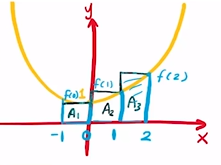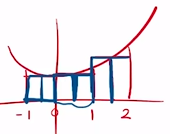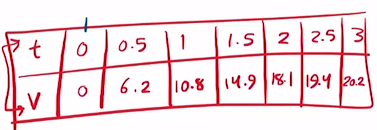Need Help?

Subscribe to Calculus A

###### \${selected_topic_name}
• Notes

5. (a) Estimate the area under the graph of $f(x)=1+x^{2}$
from $x=-1$ to $x=2$ using three rectangles and right
endpoints. Then improve your estimate by using six
rectangles. Sketch the curve and the approximating
rectangles.

(b) Repeat part (a) using left endpoints.
(c) Repeat part (a) using midpoints.
(d) From your sketches in parts (a)-(c), which appears to be
the best estimate?

$f(x)=1+x^{2}$

$\frac{2-(-1)}{3}=\frac{3}{3}=1=\Delta x$

$\Delta x=\frac{b-a}{n}$

$R_{3}=\Delta x[f(0)+f(1)+f(2)]$

$=1[1+2+5]$

$R_{3}=8$$\Delta x=\frac{b-a}{n}=\frac{2-(-1)}{6} \Rightarrow \Delta x=0.5$

$f(x)=1+x^{2}$$R_{6}=\Delta x[f(2)+f(1.5)+f(1)+f(0.5)+f(0)+f(-0.5)]$

$=0.5\left[5+\frac{13}{4}+2+5 / 4+1+5 / 4\right]=0.5(13.75)=6.875$

$f(x)=1+x^{2}$

$\Delta x=\frac{b-a}{n}=\frac{2-(-1)}{3}=1$

$L_{3}=\Delta x[f(-1)+f(0)+f(1)]=1[2+1+2] \Rightarrow L_{3}=5$

$\Delta x=\frac{b-a}{n}=\frac{2-(-1)}{6}=0.5$

$L_{6}=\Delta x[f(-1)+f(-0.5)+f(0)+f(0.5)+f(1)+f(1.5)]$

$=0.5\left[2+5 / 4+1+5 / 4+2+{13} / 4\right] \Rightarrow L_{6}=5.375$

$R_{3}=8$

$R_{6}=6.675$

$\Delta x=1 \quad \longrightarrow 0.5$

$f(x)=1+x^{2}$$\Delta x=1$

$A_{1}=\Delta x \cdot f(-0.5)$
$A_{2}=\Delta x \cdot f(0.5)$

$A_{3}=\Delta x \cdot f(1.5)$

$M_{3}=\Delta x[f(-0.5)+f(0.5)+f(1.5)]=1[5 / 4+5 / 4+13 / 4]$

$M_{3}=5.75 \longrightarrow R_{3}=8, L_{3}=5$

$\left(M_{6}\right)=\Delta x [f(-0.75)+f(-0.25)+f(0.25)+f(0.75)+f(1.25)+f(1.75)]$

$=0.5[11.875]=5.9375$

$L_{6}, R_{6}$

The speed of a runner increased steadily during the first
three seconds of a race. Her speed at half-second intervals
is given in the table. Find lower and upper estimates for
the distance that she traveled during these three seconds.

$\begin{equation} \begin{array}{|c|c|c|c|c|c|c|}\hline t(s) & {0} & {0.5} & {1.0} & {1.5} & {2.0} & {2.5} & {3.0} \\ \hline v(\mathrm{ft} / \mathrm{s}) & {0} & {6.2} & {10.8} & {14.9} & {18.1} & {19.4} & {20.2} \\ \hline\end{array} \end{equation}$$L_{6}=\Delta x[f(0)+f(0.5)+f(1)+f(1.5)+f(2)+f(2.5)]$

$L_{6}=34.7 \mathrm{ft}$ Lower estimate

$R_{6}=\Delta x[f(3)+f(2.5)+f(2)+f(1.5)+f(1)+f(0.5)]$

$R_{6}=44.8 \mathrm{ft}$ Upper estimate